# Conditional Formula

If you want to perform a calculation when a cell contains a specific value you can create a conditional formula.
A conditional formula returns one value if the condition is True and a different value if the condition is False.
A conditional formula can be created in several different ways.
There are 3 useful functions that can be used to create conditional formulas.
The IF function can return a value based on whether a certain condition is True or False.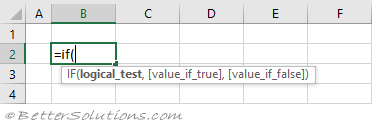The IFS function can return a value based on multiple conditions.
The SWITCH function can return a value based on a list of exact matches.

### Single Condition - IF Function

You can use any combination of cell references, operators, constants and even other functions to create this logical test.
The following Operators can be used to generate True or False values.
These operators include: greater than (>), less than (<) and equal to (=).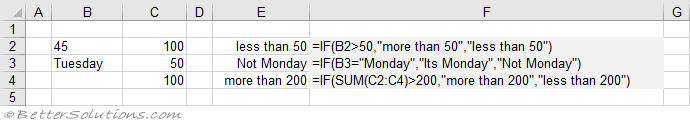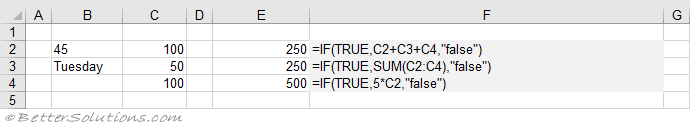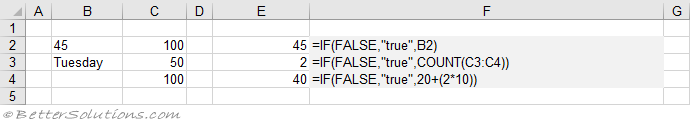### Single Condition - NOT, AND and OR Functions

These three functions are often included in the "logical_test" for slightly more advanced conditions.
Cell E3 - Return the opposite of a True or False value you would use the NOT function.
Cell E4 - Return the logical AND for two different conditions you would use the AND function.
Cell E5 - Return the logical OR for two different conditions you would use the OR function.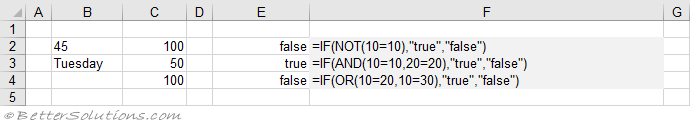### Multiple Conditions - IF Function (nested)

If you want to check for multiple conditions you can use "nested" IF functions.
When you nest the IF functions, you specify an IF function as the value of either of the "value_if_true" or "value_if_false". arguments
You can combine or nest up to 64 levels of IF functions within one formula.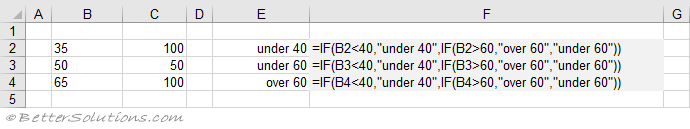### Multiple Conditions - IFS Function

The IFS function can return a value based on multiple conditions.
If you want to perform multiple conditions, instead of using nested IF functions you could use the IFS function.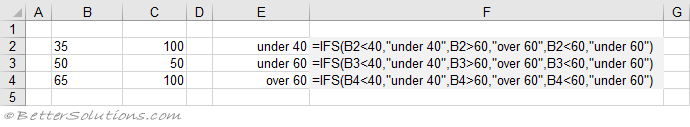### Multiple Conditions - SWITCH Function

The SWITCH function can return a value based on a list of exact matches.